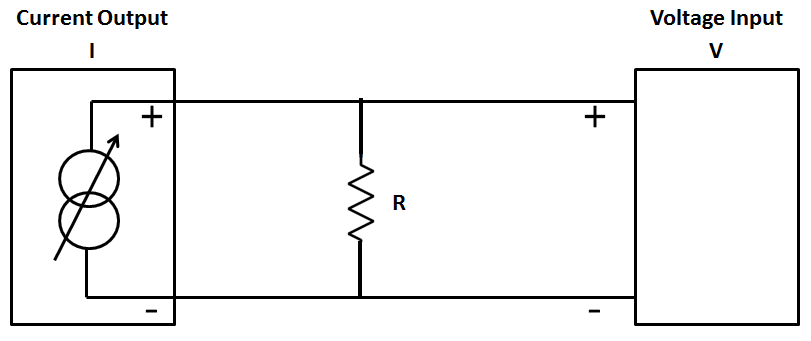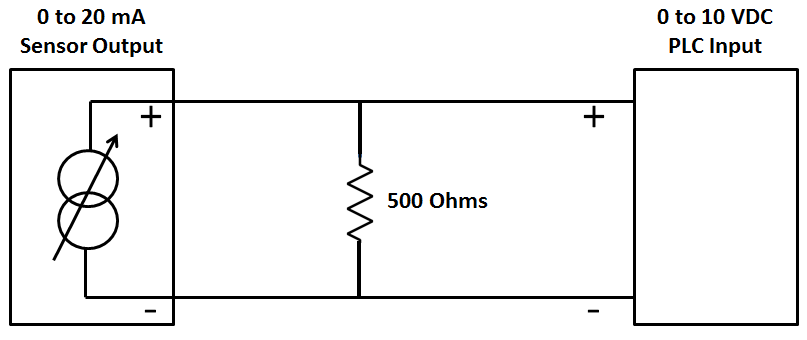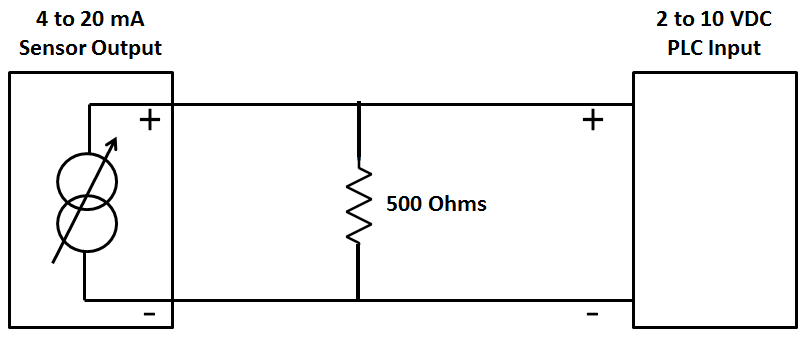Home » How to Convert Current to Voltage using Resistor ?

# How to Convert Current to Voltage using Resistor ?

In this article, we discuss about How to Convert Current to Voltage using Resistor with different examples like 0-20 mA to 0-10 VDC Conversion, 4-20 mA to 2-10 VDC Conversion, 0-20 mA to 0-5 VDC Conversion.

## Convert Current to Voltage

It is extremely simple to measure 0-20 mA signal with a device that will measure only Voltage inputs. If the Voltage input module available will accept a 0-10 Vdc signal, but may not accept a 0-20ma signal directly.

Basically, Ohms law is used to calculate a resistor value in order to convert the 0-20ma signal to a voltage.## Example : 0-20 mA to 0-10 VDC Conversion

Ohms law states: R = V/I where V is the Voltage, I is the current and R is the resistance

R = 10V/0.020A = 500 OhmsV = I*R = 0*500 = 0V

V = I*R = 0.020*500 = 10V

## Example : 4-20 mA to 2-10 VDC Conversion

Ohms law states: R = V/I where V is the Voltage, I is the current and R is the resistance

R = 10V/0.020A = 500 OhmsV = I*R = 0.004*500 = 2V

V = I*R = 0.020*500 = 10V

## Example : 0-20 mA to 0-5 VDC Conversion

Ohms law states: R = V/I where V is the Voltage, I is the current and R is the resistance

= 5V/0.020A = 250 OhmsV = I*R = 0*250        = 0V

V = I*R = 0.020*250 = 5V

Note:-

• To avoid damage you must ensure that the external current source has short-circuit protection in all conductor cases.
• The external resistor is a source of error because of its dependency on temperature and its inaccuracy.
• In order to obtain measuring results that are as precise as possible it is recommended to use resistors with tolerances that are as small as possible.

Credits : myplctechnology blog

#### Pulse Timer Instruction in PLC Programming

This website uses cookies to improve your experience. We'll assume you're ok with this, but you can opt-out if you wish. Accept Read More

WordPress Image Lightbox
Send this to a friend